# ggplot2设置坐标轴范围_ggplot2:定位## 1. 分面

ggplot2提供两种分面类型：网格型（facetgrid) 和 封装型 （facet_wrap)

mpg2 <- subset(mpg, cyl != 5 & drv %in% c("4","f"))


2.1 网格分面

（1）不进行分面，即不使用函数facet_grid或机上命令facet_null() 此时，没有行或列被分面化，我们将得到一个单独的面板。

qplot(cty,hwy,data=mpg2)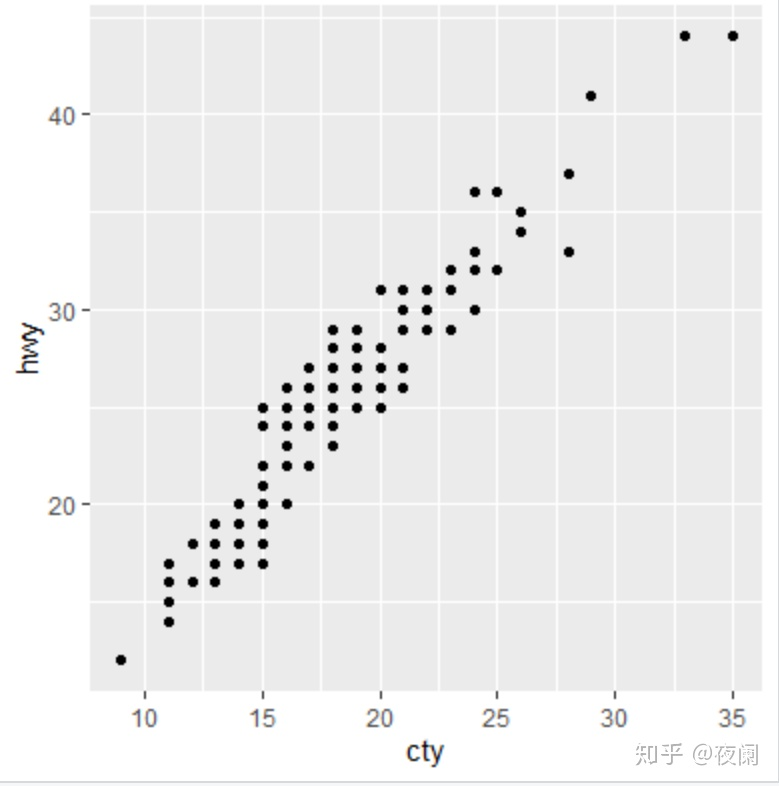（2）一行多列：“.~a"。 电脑屏幕通常较宽，因此这个方向最适合数据的展示。另外因为纵坐标相同，这个方向也有助于y位置的比较

qplot(cty,hwy,data=mpg2) + facet_grid(.~cyl)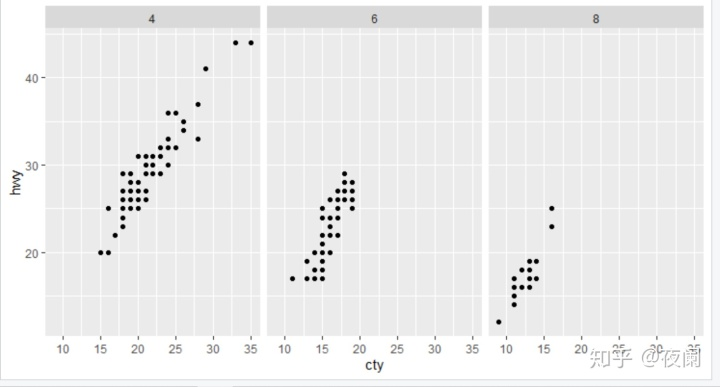（3）一列多行：”b~“。横坐标轴相同，利于x比较，尤其是对数据分布的比较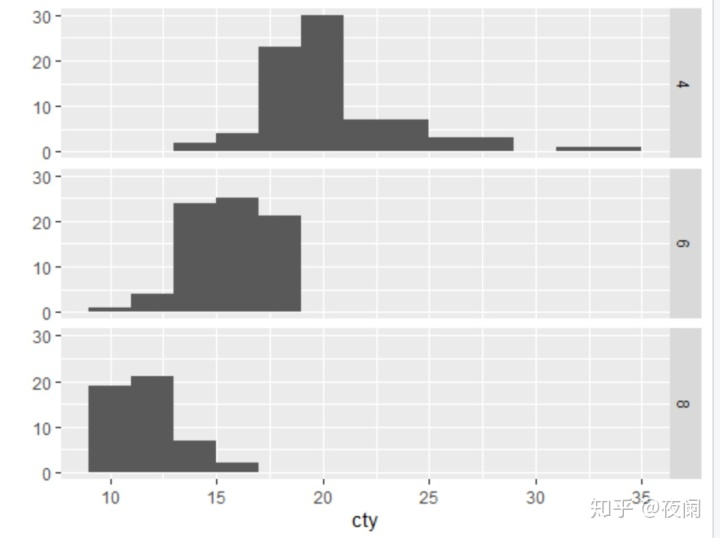（4） 多行多列：”a~b"。我们通常将因子水平数目最大的变量按列放

qplot(cty,hwy,data=mpg2)+facet_grid(drv~cyl)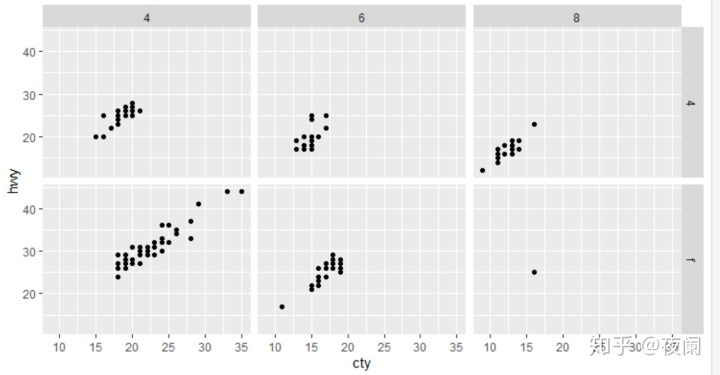（5）多个变量的多个水平在行或者列上：~a+b 或 a+b ~. 。 一般说来这种方法不怎么实用，除非变量因子水平个数比较少，或电脑屏幕很宽，或需要生成一个瘦长的海报。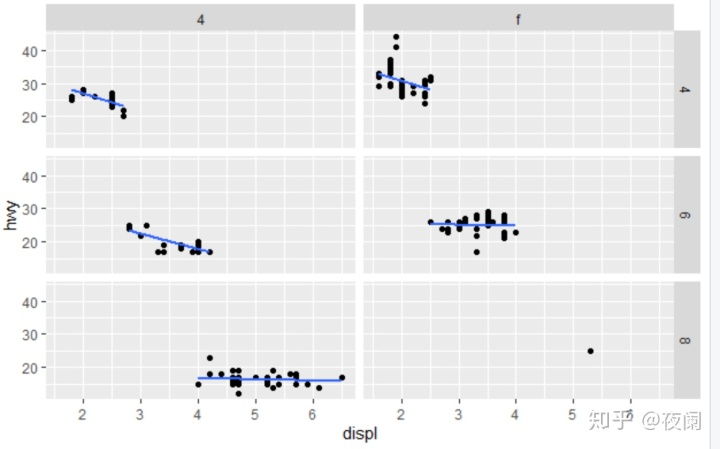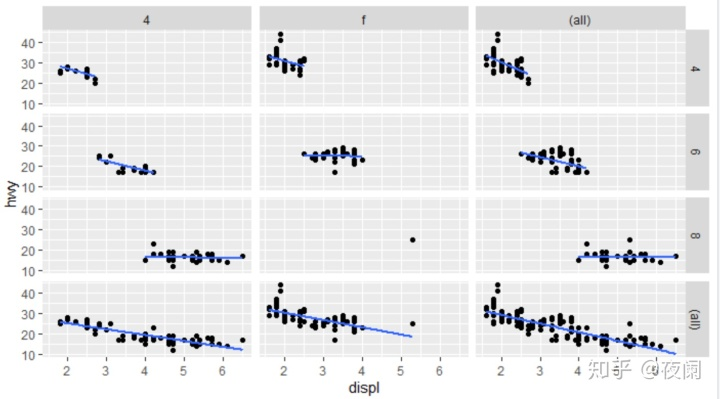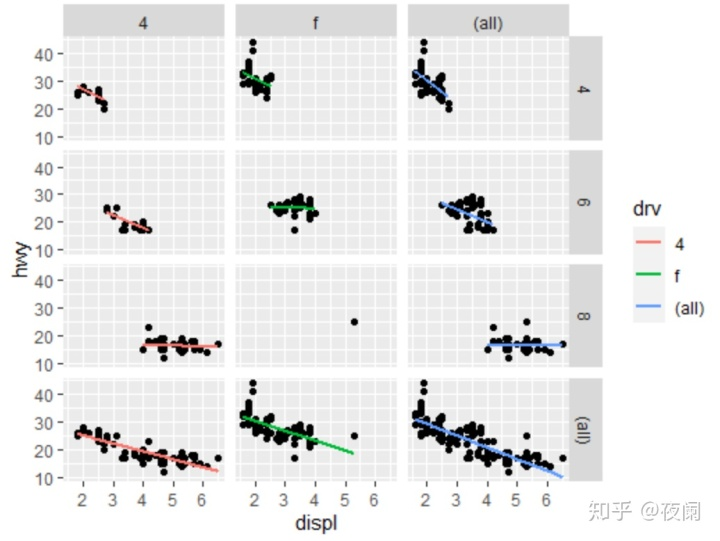## 2.2 封装分面

facet_wrap不是用两个或更多的变量来生成一个2维网格，而是先生成一个长面板，再封装

## 2.3 标度控制

scales = "fixed" /"free"

scales = "free_x" / "free_y"

library(reshape2)
em <- melt(economics,id = "date")
qplot(date,value,data = em, geom = "line", group = variable)+
facet_grid(variable ~ ., scale = "free_y")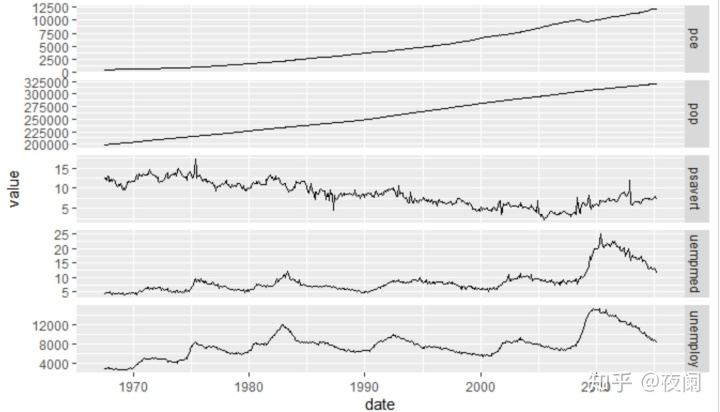facet_grid 还有一个额外的参数space，值可为”free" 或 “fixed”。当space设定为free时，每行/列与其标度范围成比例

mpg3 <- within(mpg2,{model <- reorder(model,cty)
manufacturer <- reorder(manufacturer, -cty)})
models <- qplot(cty,model,data=mpg3)
models
models + facet_grid(manufacturer ~ ., scales="free", space="free")+
theme(strip.text.y = element_text())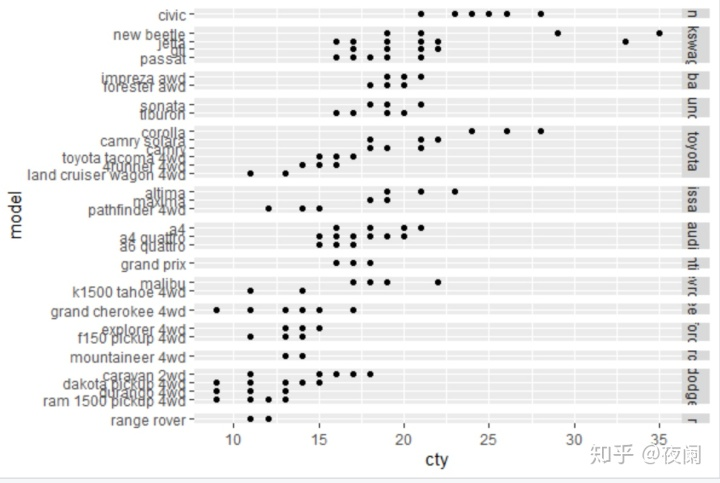## 2.5 分组与分面

xmaj <- c(0.3,0.5,1,3,5)
xmin <- as.vector(outer(1:10,10^c(-1,0)))
ymaj <- c(500,1000,5000,10000)
ymin <- as.vector(outer(1:10,10^c(2,3,4)))
dplot <- ggplot(subset(diamonds,color%in%c("D","E","G","J")),
aes(carat,price,colour=color))+
scale_x_log10(breaks=xmaj,labels=xmaj,minor=xmin)+ ##minor控制刻度
scale_y_log10(breaks=ymaj,labels=ymaj,minor=ymin)+
scale_colour_hue(limits=levels(diamonds$color))+ theme(legend.position = "none") dplot + geom_point() dplot + geom_point() + facet_grid(.~color) dplot + geom_smooth(method = lm, se =F, fullrange =T) dplot + geom_smooth(method = lm, se =F, fullrange =T)+facet_grid(.~color)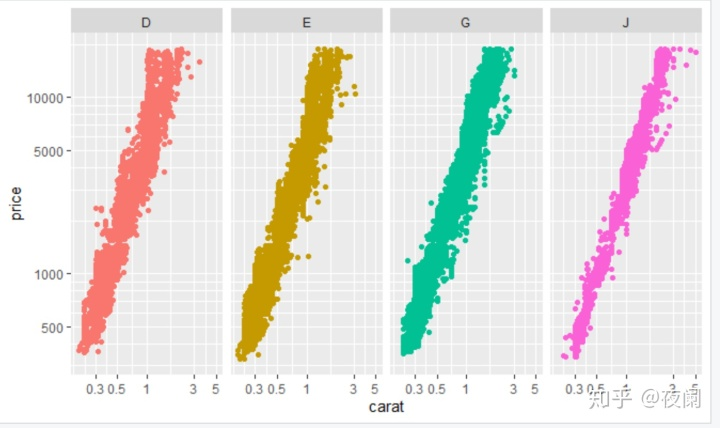## 2.6 并列与分面 原书qplot，position=dodge似乎已经被弃用，这里直接用ggplot ggplot(aes(x=color,fill=cut),data=diamonds)+ geom_bar(position="dodge2") ggplot(aes(x=cut,fill=cut),data=diamonds)+ geom_bar(position="dodge")+facet_grid(.~color)+ theme(axis.text.x=element_text(angle=90,hjust=1,size=8,colour = "grey50"))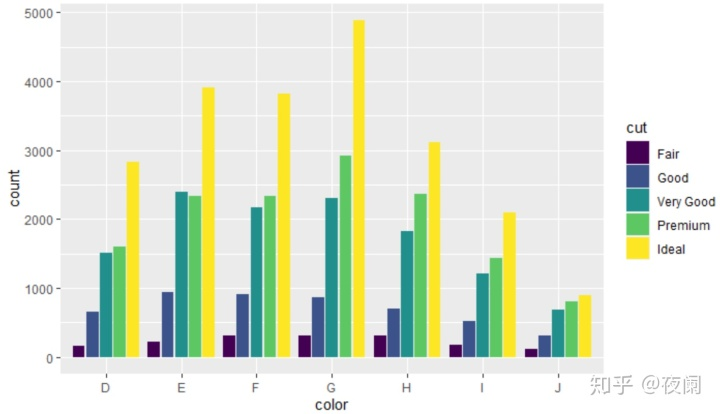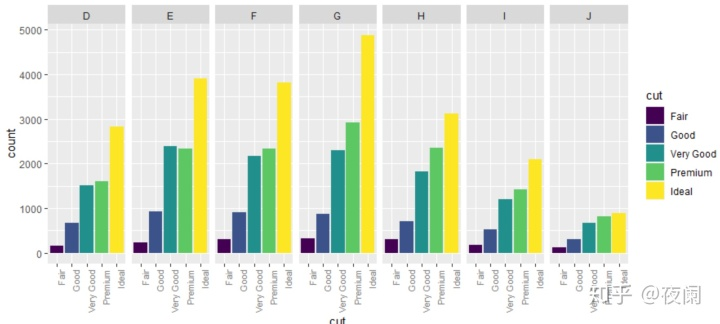mpg4 <- subset(mpg,manufacturer%in%c("audi","volkswagen","jeep")) mpg4$manufacturer <- as.character(mpg4$manufacturer) mpg4$model <- as.character(mpg4$model) base <- ggplot(mpg4,aes(fill=model))+ geom_bar(position="dodge")+ theme(legend.position = "none") base + aes(x = model)+ facet_grid(.~manufacturer) last_plot()+facet_grid(.~manufacturer,scales="free_x",space = "free") base+aes(x = manufacturer) ## 2.7 连续型变量 对连续型变量进行分面，首先需要将其变换为离散型，有三种方法： （1） 将数据分为n个长度相同的部分：用cutinterval(x, n=10) 控制划分数目， （2）或用 cut_interval(x, length=1) 控制每个部分的长度。 （3） 将数据划分为n个有相同数目点的部分：cut_number(x, n=10) mpg2$displ_ww <- cut_interval(mpg2$displ,length=1) mpg2$displ_wn <- cut_interval(mpg2$displ,n=6) mpg2$displ_nn <- cut_number(mpg2\$displ,n=6)

plot <- qplot(cty,hwy,data=mpg2)+labs(x=NULL,y=NULL)
plot + facet_wrap(~displ_ww,nrow=1)
plot + facet_wrap(~displ_wn,nrow=1)
plot + facet_wrap(~displ_nn,nrow=1)

## 3. 坐标系

coord_cartesian()

equal 同尺度笛卡尔坐标系

flip 翻转的笛卡尔坐标系

trans 变换的笛卡尔坐标系

map 地图射影

polar 极坐标系

coord_cartesian()有两个参数xlim，ylim，和limit不同，当设定笛卡尔坐标系的范围时，我们使用的仍是所有的数据，只不过只展示一小片图形区域。好比用放大镜管卡图形一样

p <- qplot(disp,wt,data=mtcars)+geom_smooth()
p + scale_x_continuous(limits = c(325,500))
p+coord_cartesian(xlim=c(325,500))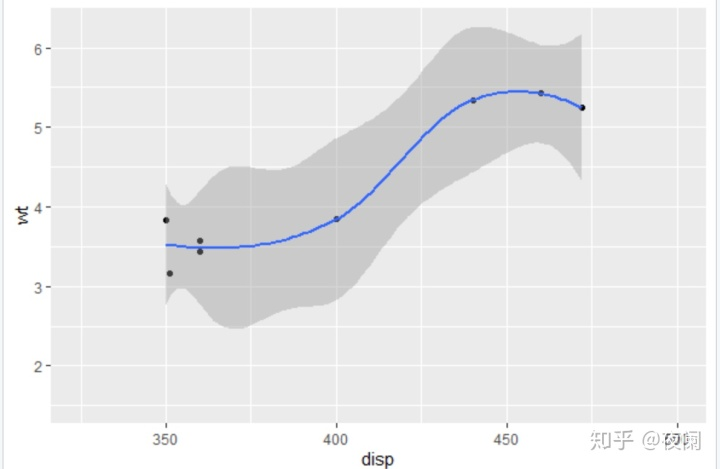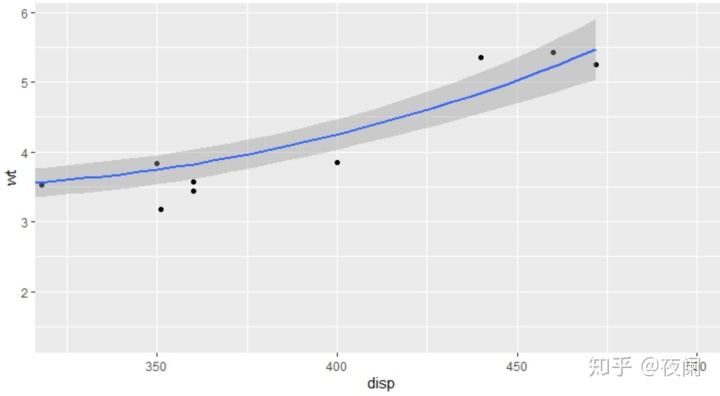d <- ggplot(diamonds,aes(carat,price))+
stat_bin2d(bins=25,colour="grey70")+
theme(legend.position = "none")
d + scale_x_continuous(limits=c(0,2))
d+coord_cartesian(xlim=c(0,2))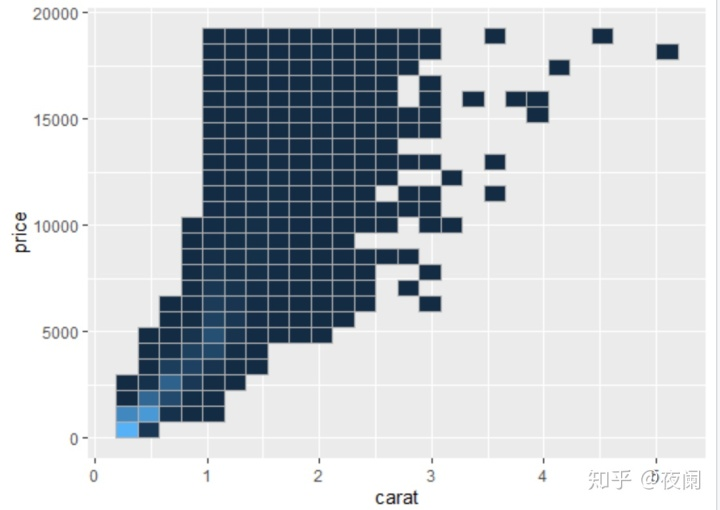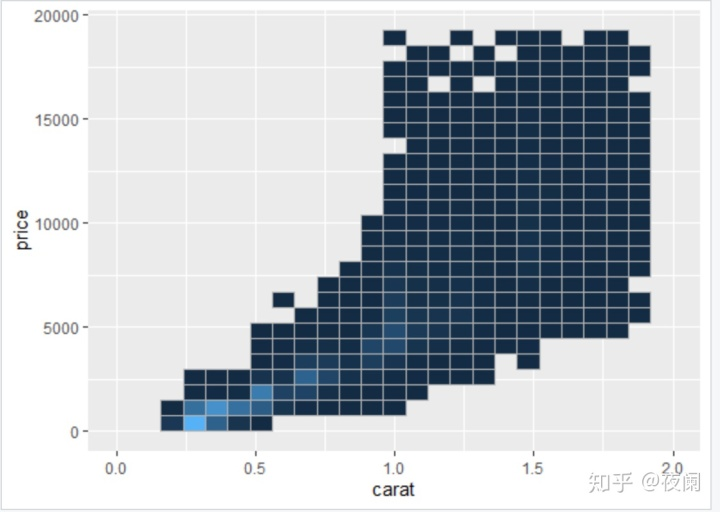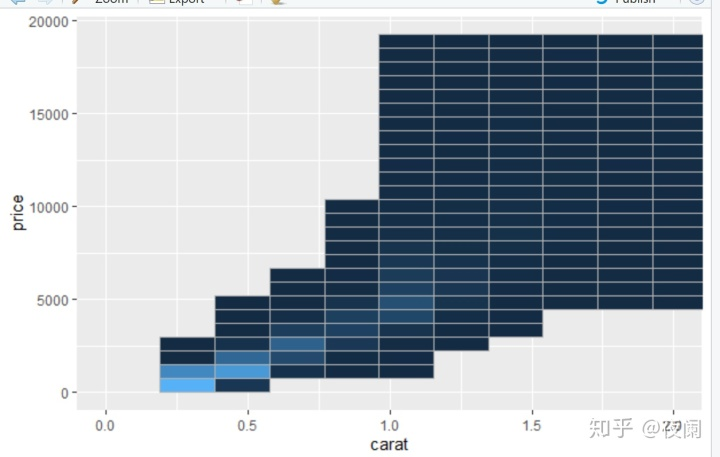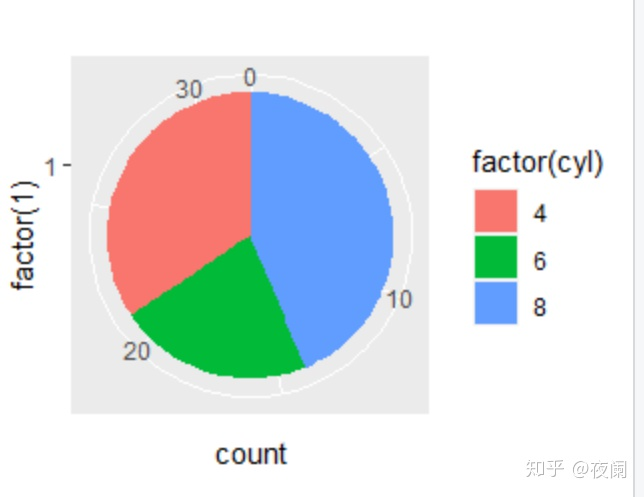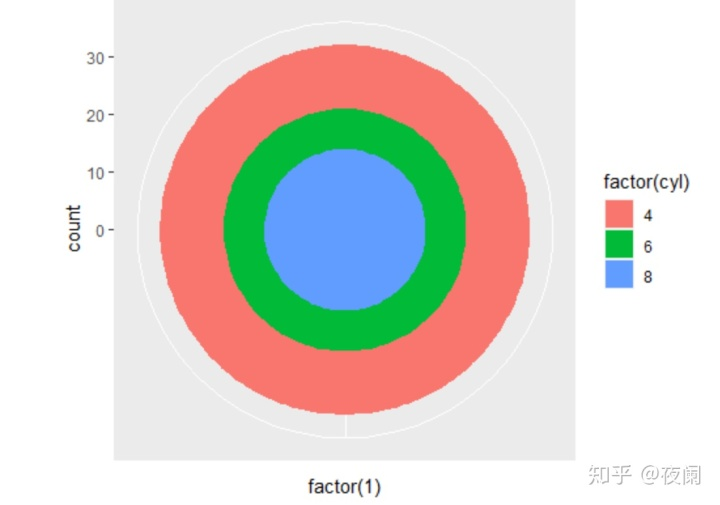11-205091
11-201万+
01-10282
11-209935
11-20959
11-203332
10-073万+
11-26455
12-201729
04-234万+
05-186284
01-113457
01-111167
07-089103
11-201371
11-20327
08-0210万+
05-214万+
11-20779

### “相关推荐”对你有帮助么？

•非常没帮助
•没帮助
•一般
•有帮助
•非常有帮助被折叠的  条评论 为什么被折叠?到【灌水乐园】发言点击重新获取扫码支付1.余额是钱包充值的虚拟货币，按照1:1的比例进行支付金额的抵扣。
2.余额无法直接购买下载，可以购买VIP、C币套餐、付费专栏及课程。余额充值